# dB and dBm for dummies

## How to easy calculate and understand dB and dBmDecibel cover made by — tartila, user3824803 and nsit0108

Decibels are something most people associate with sounds and loudness in the sense of sound pollution. But it has a lot more to offer than barely indicating a number, that informs you if the noise coming from the new washing machine is okay. Or if the new car you purchased will drive your neighbor crazy with loud noises 5 in the morning when you hit the gas pedal going off to work!

This article is produced for people, that need an effortless way to understand dB and dBm. But also, for all the other people that have some spare time, and merely want to absorb something new. Therefore, let us just dive straight into it!

# The basic behind decibels

Foremost, decibels represent a measurement that explains the ratio between two forces. It does not state anything about the specific force. Only that one force is greater than another and how much that is. Easy right. Well? let’s place it into some context.

Let us say you ask me how much do I earn on my job? I inform you that I receive twice the amount as a regular writer does. This does not state anything about my real salary, but it tells you something about the ratio. This is exactly what decibel is about. If you know what a regular writer gets paid, you can calculate my payment. The same goes for decibels, if you know the input you can also calculate the output based on the number of decibels (dB) (Mark, 2007, s. 1021).

To sum up, decibels do not represent an exact number, it only tells you the ratio between two forces.

# The heavy math behind decibels

An excellent example for you to grasp it maybe a little bit better, can be an amplifier. On one side you are transferring 2w to it, and it produces 6w for you. Decibels will here be able to tell you the ratio between 2w and 6w.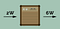2w in and 6w out by — freepik

So how do we calculate this? Decibels are made up of a logarithmic scale. This means it follows certain rules, that we will look at when we discuss dBm. But for now, you only need to know how the formula looks like.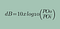dB formula by — Author

Let us break the formula down. dB represents the source in this example it was electricity. POo stands for power output, and POi represents power input. I am using POi and POo instead of Pi and Po as the regular formulae use. This is to make sure you do not think that power input (Pi) is PI as in 3.14 (Mark, 2007, s. 1025).

# Finding dB

Great, now let us utilize it with our example to see what happens. We had 2w input and 6w output. The formula will then look something like this: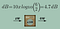Using the dB formula by — Author and freepik

The ratio between in and out is 4.7 dB. So, what happens if the output is less than the input? This can happen if you deliver some power over a wire, during the time it travels there might be some speedbumps along the way. Let’s say you send 10 mW but only get 8mW. The formula is the same.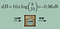Using the dB formula by — Author and freepik

Nothing changed, we nevertheless get some dB ratio. But this time in a negative direction since we are losing power.

# Using dB to find power input or output

If we want to find the power output or input, we just tweak the formula a little bit. All we do is moving dB to the right and the power sources to the left.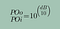Finding POo and POi by — Author

To find one value we need at least two values. Calculation of POo needs POi and DB. POi needs POo and dB. dB needs Poo and Poi. Let’s say you have some sort of power source that gives you 1 mW. You direct this through an amplifier that shows you 3 dB. How much output do you then have? Easy!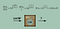Finding POo and POi by — Author and freepik

Take a moment and go back now and look at the dB formula once more before you advance. And examine the examples for finding power output. You do not need to know them by heart, merely understand the setup. dB is not an except number or anything, it is only a reference number that shows you the ratio between two forces.

# What is dBm?

You might now remember dB is a reference number, and it shows you the ratio between two forces. Well, dBm represents the opposite. dBm possesses a fixed value, POi is consistently 1. And it is used to indicate the power level in signals or other electrical equipment that gives out some sort of power. They both follow the same formula for calculation input and output, but Poi is always 1 as a reference value.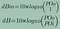dBm and dB by — Author

Since it uses the same formula we can use the same formula as earlier to find the power output based in decibel. Here are three examples to make it more simple:

Example 1: We have a sender with 80 dBm
POo = 10^(80/10) = 10⁸ mW = 10⁵ W = 100 kW

Example 2: We have a sender with 27 dBm
P = 10^(27/10) = 501.18 mW = rounding off to 500 mW

Example 3: We have a receiver with -73 dBm
P = 10^(-73/10) = 5.01 x 10 ^-8 rounding off to 5 x 10^-8

# Easy calculation with dBm

As with all other mathematical equations, you have some sort of cheat. When it comes to dBm we have what is called the 3 and 10 rule. It does not provide you with identical numbers as the established formula does, but it is more than good enough in most cases. If we look at this table: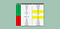Power ratio table by — Author

Each time you add 3 dBm the value is multiplied by two. And when we add 10 dBm is it multiplied by ten. We can also say that when we add 20 is it multiplied by 100. The only problem is it can be troublesome to calculate such big numbers in the head for most people. So we stick we 10 and 3 as a standard.

There are two ways to exploit this rule. One is to calculate a new output value based on an increment in dBm or to calculate the output based on a dBm value. Let us say you have 5mW, and you add 3 dBm to your current stream. What will the output then be? Yep! You are right, it goes up to 10 mW. This is because of the 3 and 10 rules. Each time you add 3, you multiply by 2!

Therefore, if you have 5 mW and you rise it with 6 dBm? Correct! That will be 20 mW!

5 mW + 6 dBm = 5 mW + 3 dBm + 3 dBm = 5 mW x 2 x 2 = 20 mW

And the identical rule applies to 10. You have 5 mW and increase it with 10 dBm. You get 50 mW! What if you add 20 dBm? Correctly, in that case, you get 500 mW.

5 mW + 20 dBm = 5 mW + 10 dBm + 10 dBm = 5 x 10 x 10 = 500 mW.

Now, let us get a little crazy what happens if you raise it with 13 dBm? You got it! You then get 100 mW. 13 is made up of 10 and 3. And that means you get x10 and x2.

5 mW + 13 dBm = 5 mW + 10 dBm + 3 dBm = 5 x 10 x 2 = 100 mW.

Every so often you subtract 10 or 3, and the identical principle will be used here as well. But instead of multiplying with 10 or 2, we dived. In summary, this is the short cheat, cheat for the rule: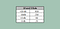10 and 3 rule by — Author

Great! Now you know how to use the 10 and 3 rule to calculate an increment in the power source based on dBm! It won’t be exact and more massive numbers will maintain a bigger gap. This is because 3 isn’t equal to 2 but to 1.995 so we round up to 2 in an effort of making it simple. But what if you purely have dBm? How can you calculate output based on dBm? This is the second way to utilize the 3 and 10 rule.

# Finding dBm with 3 and 10

The wicked trick is to find out how a value is made up of 10 and 3. Every unique number can be produced with the use of 10 and 3.

1 = 10–3–3- 3

2 = 3 + 3 + 3+ 3–10

3 = 3

4 = 10–3–3

5 = 3 + 3 +3 +3 +3–10

6 = 3 + 3

7 = 10–3

8 = 10 + 10–3–3- 3- 3

9 = 3 + 3 + 3 + 3

10 = 10

The sole rule is that you cant use the number 3 more than five times. As an example:

8 = 3 + 3 + 3 +3 +3 +3–10 is wrong since it uses more than five 3.

8 = 10 + 10–3–3–3–3 are on the other right, since it uses less then five 3.

It does not matter if it is a big or small number, they all can be made with 10 and 3 using this rule. 48 will be: 41 = 10 + 10 + 10 + 10 + 10–3–3–3. And -28 will be: -28 = -10–10–10–10 + 3 + 3 +3 +3.

Let us say you have a transmitter that is showing 6 dBm. How much energy does it generate? POi is always 1 since it is dBm. This way we recognize that we can implement the 10 and 3 rule.

6 db = 3db + 3db.

If you add 3 you multiply by 2. This gives us then:

6 db = x2 x2

And that turns into 4 mW, since we are always calculating with mW when it is dBm.

6dBm = 4 mW.

Another example is 17 dBm:

17dBm = 10 + 10 –3

10 is the same as x10 and 3 means x2. But since 3 is negative we must divide instead.

17dBm = x10 x 10 ÷2
17dBm = 50 mW

But what about negative dBm? It is the same no worries. If we use -14 dBm we get:

-14dBm = -10 -10 +3 +3

-14dBm = ÷ 10 ÷ 10 x 2 x 2

-14dBm = 4x10^-2 = 0.04 mW

This sums up the quick introduction to dB and dBm, if you enjoyed reading make sure you give this post 50 claps if you want to see more.

Do you think this was thrilling and want to read on? On that occasion I recommend you look at some of my other articles on technology. Over here are some examples you might enjoy.

# References

Mark, J. E. (2007). Physical Properties of Polymers Handbook. New York: Springer.

## Technology Hits

Important, informing, and engaging stories on technology

Written by

## Marcus Knoph

IT Consultant with extensive experience in computer security / programming, and leadership / management development of personnell.

## Technology Hits

Important, high-impact, informative, and engaging stories on all aspects of technology.

Written by

## Marcus Knoph

IT Consultant with extensive experience in computer security / programming, and leadership / management development of personnell.## Technology Hits

Important, high-impact, informative, and engaging stories on all aspects of technology.

Medium is an open platform where 170 million readers come to find insightful and dynamic thinking. Here, expert and undiscovered voices alike dive into the heart of any topic and bring new ideas to the surface. Learn more

Follow the writers, publications, and topics that matter to you, and you’ll see them on your homepage and in your inbox. Explore

If you have a story to tell, knowledge to share, or a perspective to offer — welcome home. It’s easy and free to post your thinking on any topic. Write on Medium